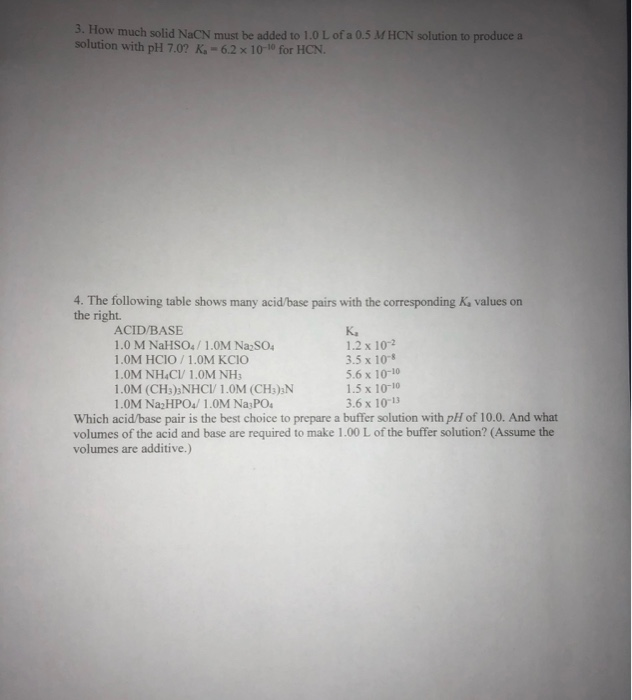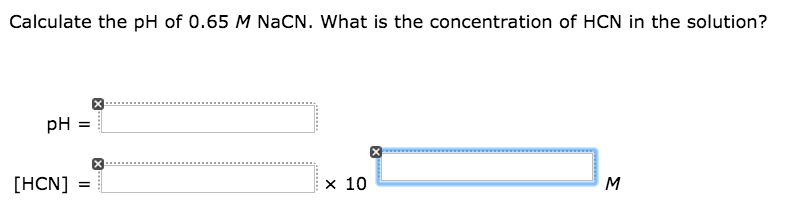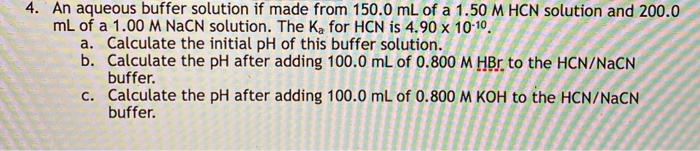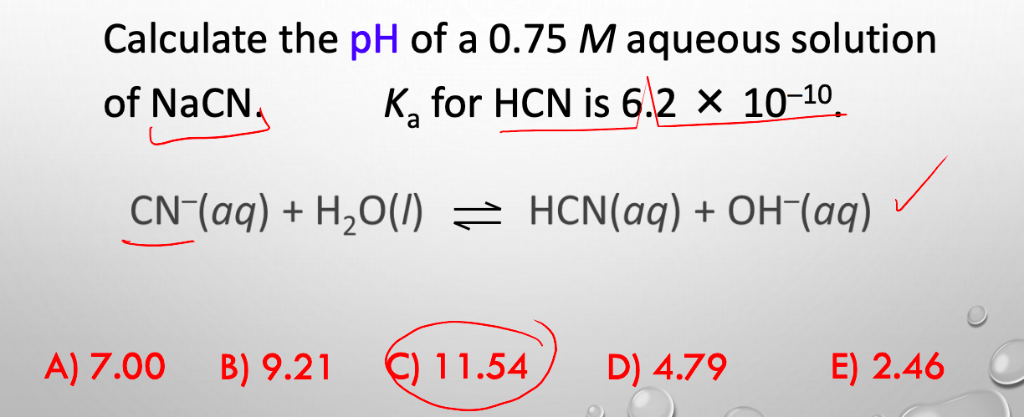Question

# What concentration of NaCN must be added to a 0.5 M HCN solution to produce a...

What concentration of NaCN must be added to a 0.5 M HCN solution to produce a buffer solution with pH 7.0? Ka = 6.2 x 10–10 for HCN

a) 3.3 M

b) 0.49 M

c) 6.9 x 10-5 M

d) 0.0031 M

e) 0.22 M

To solve this we need to use Henderson-Hasselbalch equation which is given by,

pH = pKa + log([base]/[acid])

Since Ka = 6.2 * 10-10

Therrefore pKa = -log(Ka) = -log(6.2 * 10-10) = 9.2076

Therefore

7.0 = 9.2076 + log([base]/0.5)

7.0 - 9.2076 = log([base]/0.5)

-2.2076 = log([base]/0.5)

Take antilogarithm on both side

10-2.2076 = ([base]/0.5)

0.0062 = ([base]/0.5)

Multiply both side by 0.5

0.0062 * 0.5 = [base]

0.0031 M = [base]

Therefore concentration of NaCN added to make buffer solution is 0.0031 M.

If you find any mistake, please mention in the comment box. Thanks.

#### Earn Coins

Coins can be redeemed for fabulous gifts.

Similar Homework Help Questions
• ### 3. How much solid NaCN must be added to 1.0 L of a 0.5 MHCN solution...3. How much solid NaCN must be added to 1.0 L of a 0.5 MHCN solution to produce a solution with pH 7.0? K. - 6.2 x 10- for HCN. K 4. The following table shows many acid/base pairs with the corresponding K, values on the right. ACID/BASE 1.0 M NaHSO4/ 1.0M Na2SO4 1.2 x 10-2 1.0M HCIO/ 1.0M KCIO 3.5 x 10- 1.0M NH4Cl 1,0M NHI 5.6 x 10-10 1.0M (CH3)2NHCI/1.0M (CH3)2N 1.5 x 10-10 1.0M Na2HPO4/ 1.0M Na...

• ### 1. A buffer solution is 0.313 M in HCN and 0.220 M in NaCN . If...

1. A buffer solution is 0.313 M in HCN and 0.220 M in NaCN . If Ka for HCN is 4.0×10-10, what is the pH of this buffer solution? 2. A buffer solution is 0.473 M in H2C204 and 0.366 M in KHC2O4 . If Ka1 for H2C2O4 is 5.9*10^-2, what is the pH of this buffer solution? 3. A buffer solution is 0.392 M in NaHC2O4 and 0.329M in NaC2O4 . If Ka for HC2O4- is 6.4*10^-5, what is...

• ### Calculate the following: a. The pH of a 500.0 mL buffer solution containing 0.75 M HCN...

Calculate the following: a. The pH of a 500.0 mL buffer solution containing 0.75 M HCN (Ka = 6.2 x 10^-10) and 0.55 M NaCN b. The pH of the above buffer after the addition of 100.0 mL of 1.0 M NaOH. c. The pH of the buffer if 100.0 mL of 1.0 M HCl was added to the solution in part a.

• ### You wish to make a buffer solution at a pH 9.56 with the weak acid HCN...

You wish to make a buffer solution at a pH 9.56 with the weak acid HCN (Ka = 6.9 x 10-10 ) and its salt, NaCN..What ratio of the concentration of conjugate base to the acid: ([A- ]:[HA]) is required to attain the desired pH? a. [A- ] is 2.5 × that of [HA] b. [A- ] is 5 × that of [HA] c. [A- ] is 1 × that of [HA] d. [A- ] is ½ × that of...

• ### Calculate the pH of 0.65 M NaCN. What is the concentration of HCN in the solution?...Calculate the pH of 0.65 M NaCN. What is the concentration of HCN in the solution? PH= [HCN]= Times 10 M

• ### 4. An aqueous buffer solution if made from 150.0 mL of a 1.50 M HCN solution...4. An aqueous buffer solution if made from 150.0 mL of a 1.50 M HCN solution and 200.0 mL of a 1.00 M NaCN solution. The Ka for HCN is 4.90 x 10-10. a. Calculate the initial pH of this buffer solution. b. Calculate the pH after adding 100.0 mL of 0.800 M HBr to the HCN/NaCN buffer. c. Calculate the pH after adding 100.0 mL of 0.800 M KOH to the HCN/NaCN buffer.

• ### When NaOH is added to a buffer solution that contains 0.25 M HCN and 0.25 M...

When NaOH is added to a buffer solution that contains 0.25 M HCN and 0.25 M NaCN, which component reacts with the added hydroxide ion to maintain the pH of the solution? a) HCN - b) CN - c) Na + d) H2O e) OH -

• ### What is the pH of a 2.50 molar solution of NaCN(aq)? KA (HCN) = 6.2×10^-10 a....

What is the pH of a 2.50 molar solution of NaCN(aq)? KA (HCN) = 6.2×10^-10 a. 11.80 b. 9.60 c. 9.21 d. 4.40 e. 2.20 A. is the correct answer

• ### Calculate the pH of a 0.75M aqueous solution of NaCN, Ka for HCN = 6.2E-10 Can...Calculate the pH of a 0.75M aqueous solution of NaCN, Ka for HCN = 6.2E-10 Can someone explain to me how to do this problem step by step, and also explain why the reaction equation was written the way it was?? Calculate the pH of a 0.75 M aqueous solution of NaCN, K, for HCN is 6.2 X 1010. CNag)+ H2)HCNa)+ OH (aq) A/700 8) 9,21 15 0)479 A) 7.00 B) 9.2 C) 11.54D) 4.79 E) 2.46

• ### 10.0 g of a mixture of NaCl(s) and NaCN(s) are added to 1.00L of water. The...

10.0 g of a mixture of NaCl(s) and NaCN(s) are added to 1.00L of water. The change in volume is negligible. Once the solids have dissolved, the pH of the solution is 11.09. Therefore the percentage by mass of the mixture that is NaCN(s) is Ka (HCN) = 6.2 x 10-10 A. 36 B. 56 C. 66 D. 76 E. 46